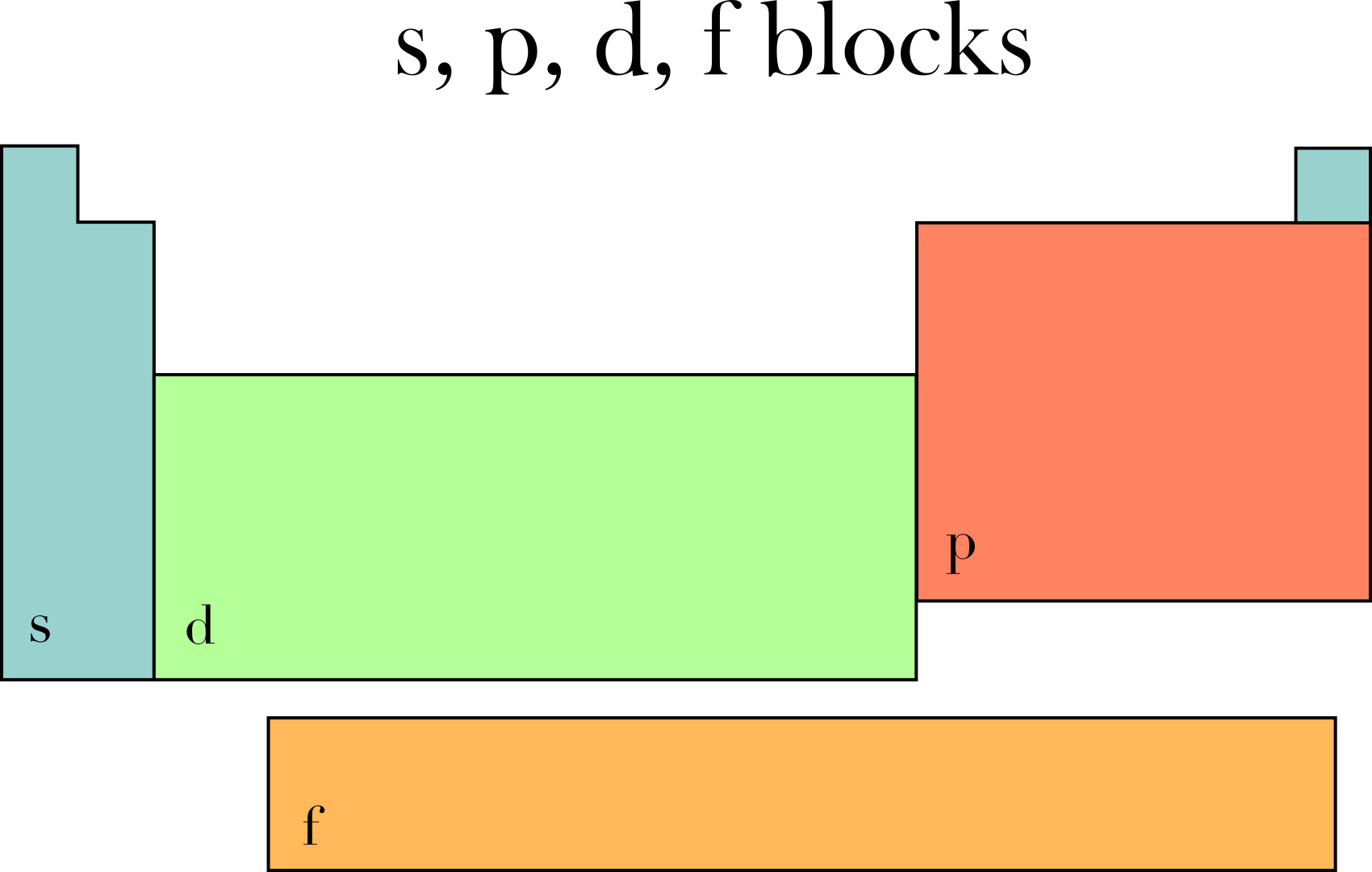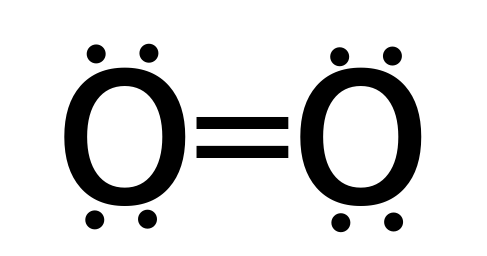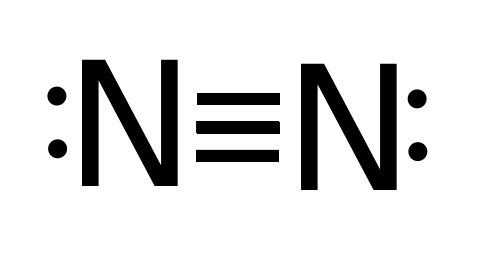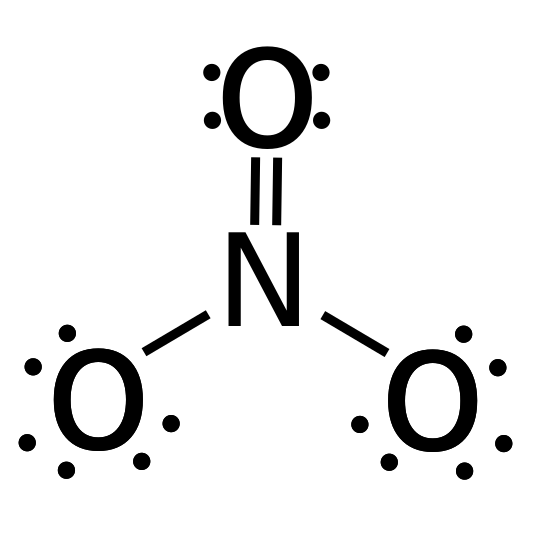# Bond Order Calculator

Created by Julia Żuławińska
Reviewed by Bogna Szyk and Jack Bowater
Last updated: Jan 20, 2020

The molecular orbital theory has never been so clear as with our bond order calculator. In the following article, we will explain what bond order is, and how to use the bond order formula. You will also find out the difference between bonding and antibonding electrons. Sounds interesting? Let's dive in!

If you're reading this, you probably already know the structure of an atom. If you don't, before you read on, check out our atom calculator - it's an excellent introduction to the topic.

## What is bond order?

The bond's order determines the stability of a molecule or ion. The higher the bond order, the stronger is the bond, thus the higher the bond energy. Also, for diatomic molecules, a greater bond order means shorter bond length.

There are a number of bond order definitions. According to valence bond theory, it is

the number of bonded electron pairs between two atoms.

In molecular orbital theory, bond order is

the difference between the number of bonding electrons and the number of antibonding electrons divided by 2.

What's the difference? Both of these theories refer to the position of electrons. While the first one is rather simple, the second one comes from quantum mechanics. We will try to explain it as simply as possible.

Our calculator uses the bond order formula from molecular orbital theory. In the next paragraph, we will explain how to use it. At the end of the text, you can also read about how to find bond order using Lewis structures.

## Bond order formula - molecular orbital theory

In molecular orbital theory, you calculate bond order using functions that describe the state of electrons in a particle. Those mathematical functions are called orbitals. In other words, the orbitals represent the most likely locations of electrons in the electron cloud, which are described using the letters: s, p, d and f. Each orbital has a particular shape and size (s is a sphere, p is in a figure of eight), and they each have a respective bond energy. We can further distinguish bonding, antibonding, and non-bonding orbitals, depending on the geometry of the bond.

To find bond order, we must determine the number of electrons in the valence shell, and the block that the molecule belongs to. To do that, look at the image shown below and compare it with the periodic table.• Block s: the number of electrons in the valence shell is equal to the element's group (except helium, which has two valence electrons)
• Block p: the number of electrons in the valence shell is equal to the element's group minus ten
• Elements from blocks d and f don't have a straightforward principle, so it would be best to check how electrons fill orbitals and work it out from there.

Electrons fill the orbitals from s to f, so from the most to least energy-efficient. Elements in the s block have one bonding and one antibonding orbital free for bonding, each with space for two electrons. Those in the p block have three orbitals that can participate in bonding, the d block - five, and for f - seven. Each orbital a bonding and an antibonding orbital, with the all of the bonding orbitals of an energy level being filled first.

How to calculate bond order? After you determine how many bonding and antibonding electrons there are, use this bond order formula:

Bond Order = (Number of bonding electrons - Number of antibonding electrons) / 2

If you're still not sure how to find bond order, don't worry - we will give you a couple of examples.

## How to calculate bond order? - examples

1. The bond order of H2 (hydrogen)

Bond order = (2 - 0) / 2 = 1

Each hydrogen atom has one valence electron. So, when two atoms bond, there are two electrons in total. They fill out the bonding orbital, as it's more energy-efficient.

1. The bond order of CO (carbon monoxide)

Bond order = (8 - 2) / 2 = 3

Carbon has two electrons in the s orbital, and two in the p orbital. Oxygen has two electrons in the s orbital, and four in the p orbital. When they bond, there are four electrons in the s orbital and six electrons on p orbital. So, in orbital s there are two bonding electrons and two antibonding electrons, and in orbital p: six bonding electrons. As the s orbitals cancel each other out, the 6 bonding orbitals form 3 bonds, which is why carbon monoxide has a triple bond!

## How to find bond order? - valence bond theory

Let's remind ourselves what is bond order once again. The bond order is equal to the number of chemical bonds between two atoms. If between two atoms there is a single bond - the bond order is one. If there's a double bond, bond order is two, and so on. To determine bond order, all you have to do is draw the Lewis structure of a molecule and establish what type of bond is between the atoms. Let's look at some examples:

1. The bond order of O2 (oxygen) - oxygen molecule has a double bond, so bond order is equal two.

Bond order = number of bonds = 21. The bond order of N2 (nitrogen) - nitrogen molecule has a triple bond, so bond order is three.

Bond order = 3If you want to find bond order for a non diatomic molecules, you can do that by finding the average of bonds between pairs of atoms. For example:

1. The bond order of NO3 (nitrate) - There's a total of four bonds and three bond groups in nitrate.

Bond order = number of bonds / number of groups = 4 / 3 = 1.33While bond order calculations are easy, finding bonding and antibonding electrons might get tricky! Come back to our bond order calculator whenever you need a quick reminder on how to calculate bond order. And before you go, check out this cell EMF calculator!

Julia Żuławińska
Bonding electrons
Antibonding electrons
Bond order
People also viewed…

### Beer-Lambert law

Use Omni's Beer-Lambert law calculator to calculate the absorbance of light as it passes through any substance. You can also use this calculator to determine the concentration of a solution using Beer's law.

### Christmas tree

Welcome to the Christmas tree calculator, where you will find how to decorate your Christmas tree in the best way. Take a look at the perfect Christmas tree formula prepared by math professors and improved by physicists. Plan in advance how many lights and decorations you'll need!

### Free fall

Our free fall calculator can find the velocity of a falling object and the height it drops from.

### Langmuir isotherm

Langmuir isotherm calculator is a simple way to compute the fraction of adsorbent surface covered by adsorbate, according to the Langmuir adsorption model.